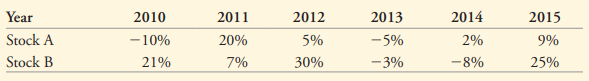### Create an Account

Home / Questions / Using the data in the following table estimate a the average return and volatility for eac...

# Using the data in the following table estimate a the average return and volatility for each stock b the covariance between the stocks and c the correlation between these two stocks

Using the data in the following table, estimate (a) the average return and volatility for each stock, (b) the covariance between the stocks, and (c) the correlation between these two stocks.Jun 08 2021 View more View LessGet Solution